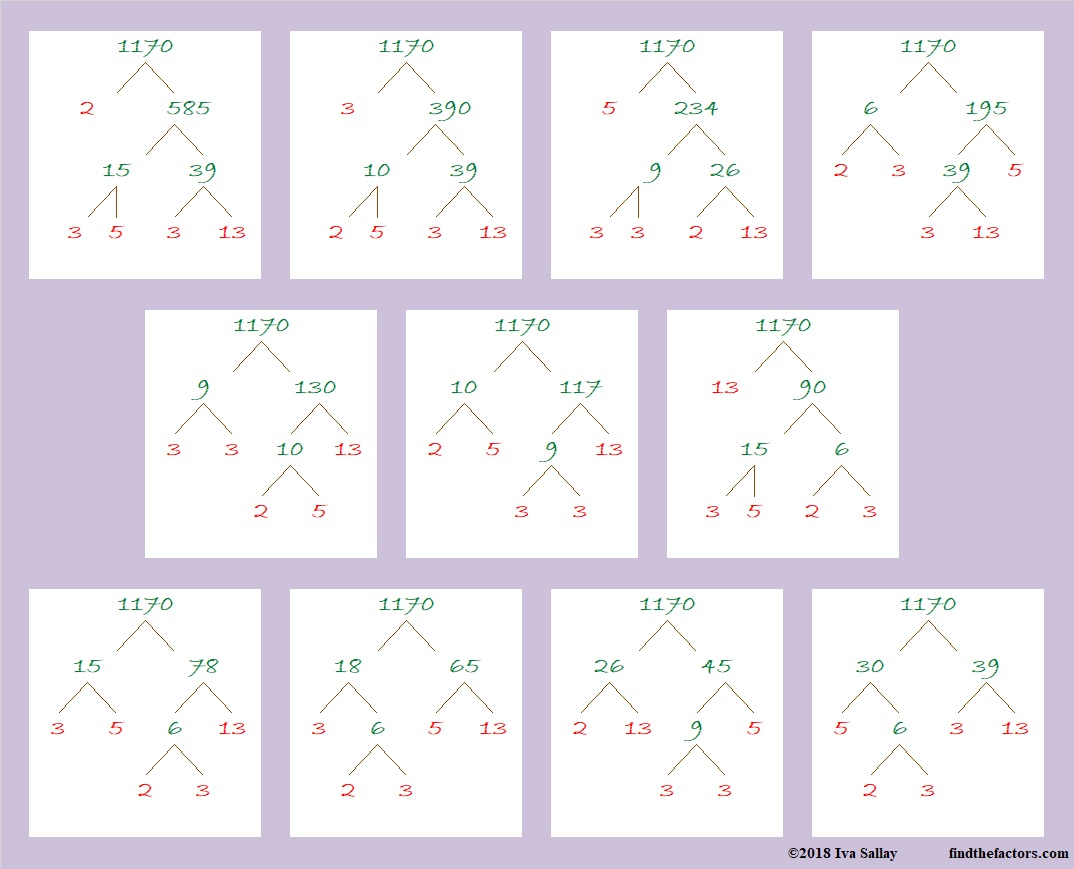# 1170 Factor Trees

1170 is one of those numbers with twenty-four factors. Why does it have so many? Because of its prime factorization. You can find a number’s prime factorization by making a factor tree. Here are eleven different factor trees for 1170. Each one of them produces the same prime factorization: 1170 = 2 × 3² × 5 × 13• 1170 is a composite number.
• Prime factorization: 1170 = 2 × 3 × 3 × 5 × 13, which can be written 1170 = 2 × 3² × 5 × 13
• The exponents in the prime factorization are 1, 2, 1, and 1. Adding one to each and multiplying we get (1 + 1)(2 + 1)(1 + 1)(1 + 1) = 2 × 3 × 2 × 2 = 24. Therefore 1170 has exactly 24 factors.
• Factors of 1170: 1, 2, 3, 5, 6, 9, 10, 13, 15, 18, 26, 30, 39, 45, 65, 78, 90, 117, 130, 195, 234, 390, 585, 1170
• Factor pairs: 1170 = 1 × 1170, 2 × 585, 3 × 390, 5 × 234, 6 × 195, 9 × 130, 10 × 117, 13 × 90, 15 × 78, 18 × 65, 26 × 45, or 30 × 39
• Taking the factor pair with the largest square number factor, we get √1170 = (√9)(√130) = 3√130 ≈ 34.2052633² +  9² = 1170
27² +  21² = 1170

1170 is also the hypotenuse of FOUR Pythagorean triples:
288-1134-1170 calculated from 27² –  21², 2(27)(21), 27² +  21²
450-1080-1170 which is (5-12-13) times 90
594-1008-1170 calculated from 2(33)( 9), 33² –  9², 33² +  9²
702-936-1170 which is (3-4-5) times 234

1170 is 102102 in BASE 4 because 4⁵ + 2(4³) +4² + 2(1) = 1170,
and it’s repdigit 2222 in BASE 8 because 2(8³ + 8² + 8¹ + 8⁰) = 2(585) = 1170